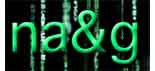Again I
spend the whole morning preparing my talks for tomorrow in the master
class. Here is an outline of what I will cover :
– examples of
noncommutative points and curves. Grothendieck’s characterization of
commutative regular algebras by the lifting property and a proof that
this lifting property in the category alg of all l-algebras is
equivalent to being a noncommutative curve (using the construction of a
generic square-zero extension).
– definition of the affine
scheme rep(n,A) of all n-dimensional representations (as always,
l is still arbitrary) and a proof that these schemes are smooth
using the universal property of k(rep(n,A)) (via generic
matrices).
– whereas rep(n,A) is smooth it is in general
a disjoint union of its irreducible components and one can use the
sum-map to define a semigroup structure on these components when
l is algebraically closed. I’ll give some examples of this
semigroup and outline how the construction can be extended over
arbitrary basefields (via a cocommutative coalgebra).

definition of the Euler-form on rep A, all finite dimensional
representations. Outline of the main steps involved in showing that the
Euler-form defines a bilinear form on the connected component semigroup
when l is algebraically closed (using Jordan-Holder sequences and
upper-semicontinuity results).

After tomorrow’s
lectures I hope you are prepared for the mini-course by Markus Reineke on non-commutative Hilbert schemes
next week.

Published in featured